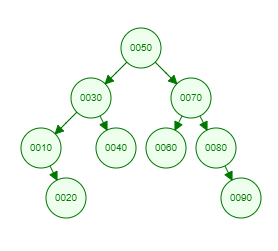Recent Tutorials and Articles
Implementing Post-Order Traversal of Binary Tree in Java
Published on: 27th October 2018

This tutorial provides code for implementing post-order traversal of a Binary Tree.

# Post-Order Traversal of Binary Tree

Binary Tree traversal is a process of visiting each node of Binary Tree exactly once. Post-Order traversal falls into depth-first search as search is deepended on child nodes of a node before moving to its sibling.

Post-order traversal is a special type of Tree traversal wherein left child and right child are visited before a node. This results into recursive function calls as you can't print a node data unless all of its left and right child nodes data have been printed.

Let's consider below Binary Tree -Here is the Post-Order Traversal of above tree -

``````20 10 40 30 60 90 80 70 50
``````

# Java Implementation of Post-Order Traversal of Binary Tree

Here is Java code for Binary Tree containing methods for inserting data and post-order traversal -

``````package com.aksain.data.structures;

public class BinaryTreeWithPostOrderTraversal<T extends Comparable<T>> {
private Node root;

private class Node {
T data;
Node left;
Node right;

private Node(T data) {
this.data = data;
}
}

private Node doInsert(T data, Node node) {
if(node == null) {
return new Node(data);
}
int result = data.compareTo(node.data);
if(result < 0) {
node.left = doInsert(data, node.left);
} else if(result > 0) {
node.right = doInsert(data, node.right);
}

return node;
}

public void insert(T data) {
root = doInsert(data, root);
}

private void doPostOrder(Node node) {
if(node == null) {
return;
}
doPostOrder(node.left);
doPostOrder(node.right);
System.out.print(node.data + " ");
}

public void postOrder() {
doPostOrder(root);
System.out.println();
}

public static void main(String[] args) {
final BinaryTreeWithPostOrderTraversal<Integer> binaryTree = new BinaryTreeWithPostOrderTraversal<>();
binaryTree.insert(50);
binaryTree.insert(30);
binaryTree.insert(10);
binaryTree.insert(20);
binaryTree.insert(40);
binaryTree.insert(70);
binaryTree.insert(60);
binaryTree.insert(80);
binaryTree.insert(90);

binaryTree.postOrder();
}
}
``````

Here is the output of above code -

``````20 10 40 30 60 90 80 70 50
``````

Thank you for reading through the tutorial. In case of any feedback/questions/concerns, you can communicate same to us through your comments and we shall get back to you as soon as possible.

Published on: 27th October 2018